Скачать презентацию CHAPTER 18 Equity Valuation Models Investments 8 th

76edb6349b4edfc88b4a803493757630.ppt

• Количество слайдов: 42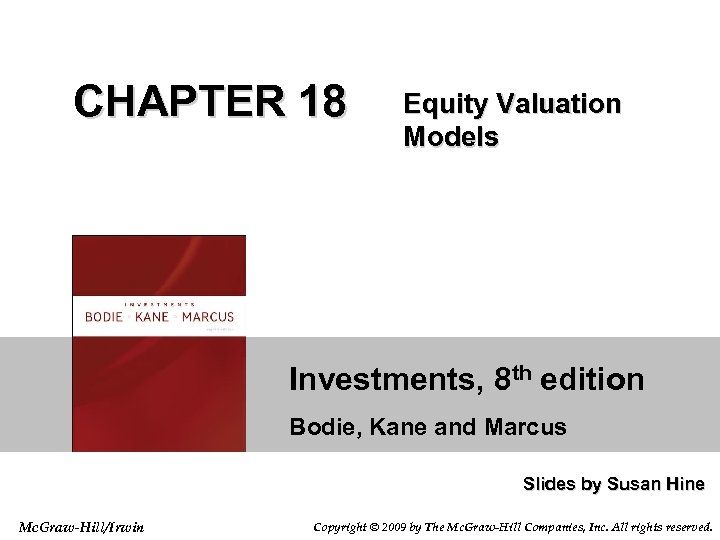CHAPTER 18 Equity Valuation Models Investments, 8 th edition Bodie, Kane and Marcus Slides by Susan Hine Mc. Graw-Hill/Irwin Copyright © 2009 by The Mc. Graw-Hill Companies, Inc. All rights reserved.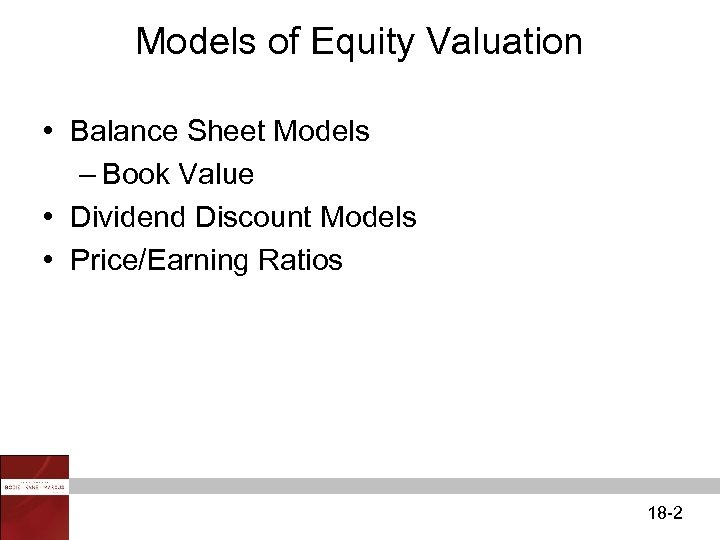Models of Equity Valuation • Balance Sheet Models – Book Value • Dividend Discount Models • Price/Earning Ratios 18 -2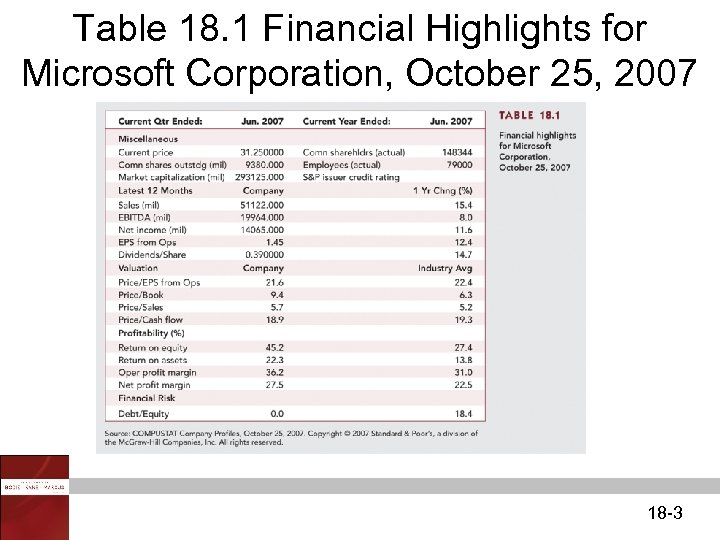Table 18. 1 Financial Highlights for Microsoft Corporation, October 25, 2007 18 -3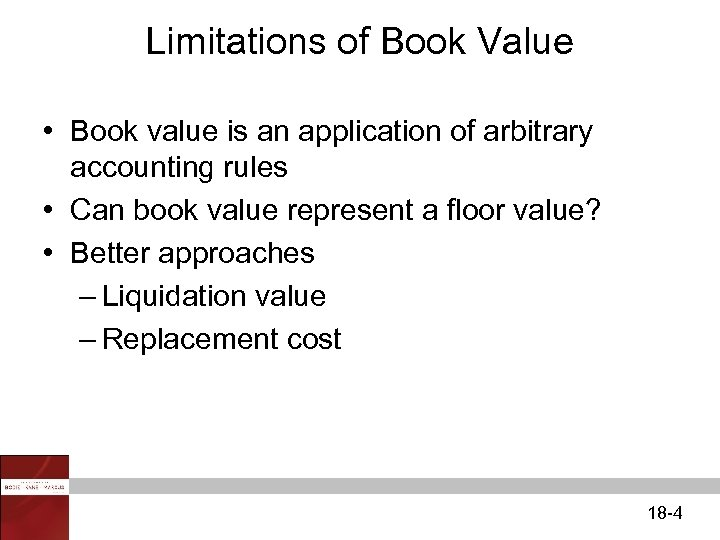Limitations of Book Value • Book value is an application of arbitrary accounting rules • Can book value represent a floor value? • Better approaches – Liquidation value – Replacement cost 18 -4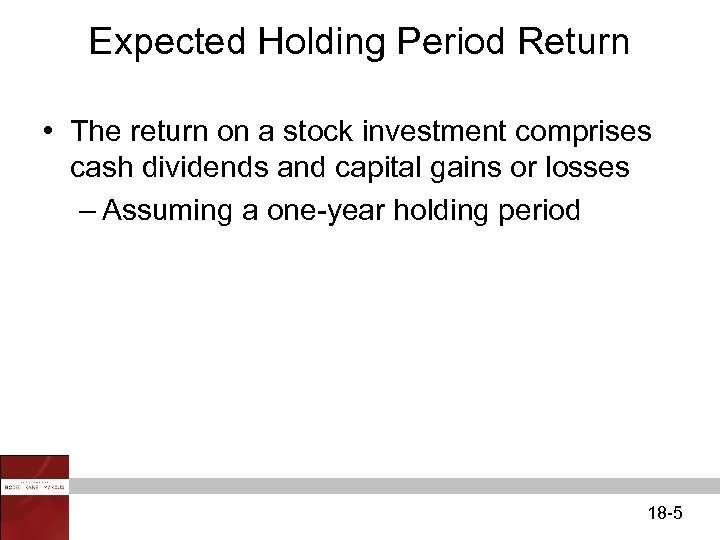Expected Holding Period Return • The return on a stock investment comprises cash dividends and capital gains or losses – Assuming a one-year holding period 18 -5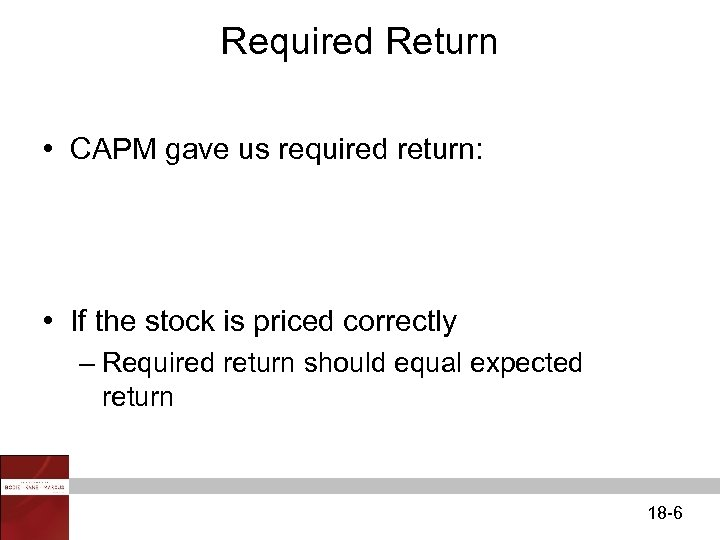Required Return • CAPM gave us required return: • If the stock is priced correctly – Required return should equal expected return 18 -6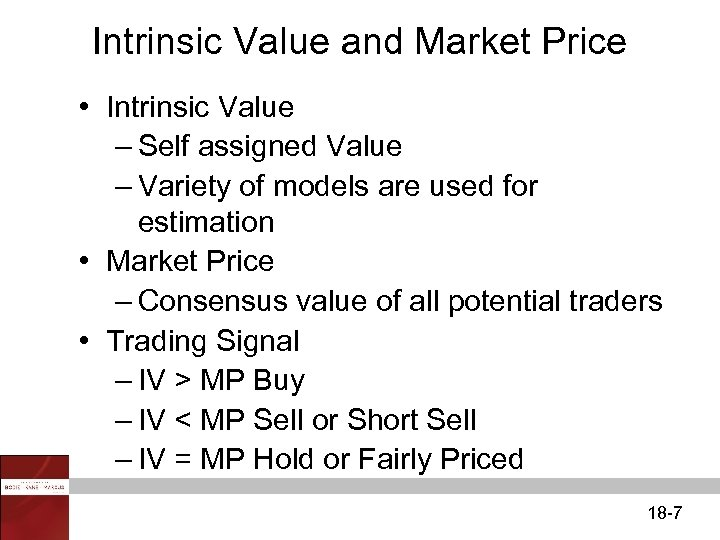Intrinsic Value and Market Price • Intrinsic Value – Self assigned Value – Variety of models are used for estimation • Market Price – Consensus value of all potential traders • Trading Signal – IV > MP Buy – IV < MP Sell or Short Sell – IV = MP Hold or Fairly Priced 18 -7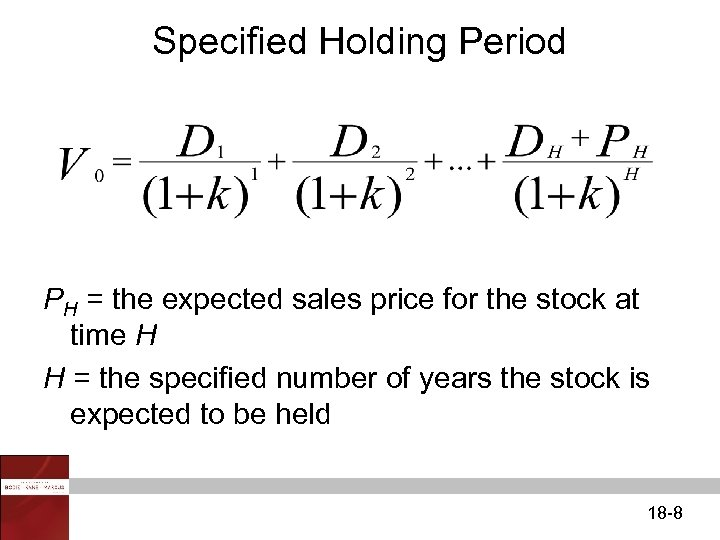Specified Holding Period PH = the expected sales price for the stock at time H H = the specified number of years the stock is expected to be held 18 -8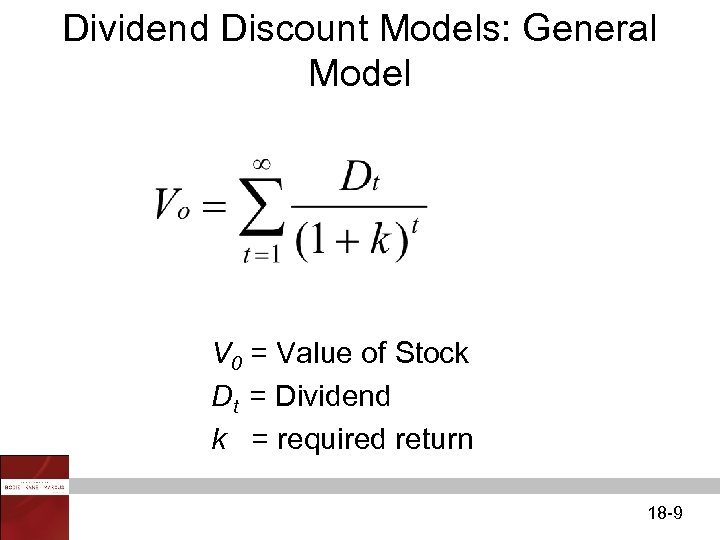Dividend Discount Models: General Model V 0 = Value of Stock Dt = Dividend k = required return 18 -9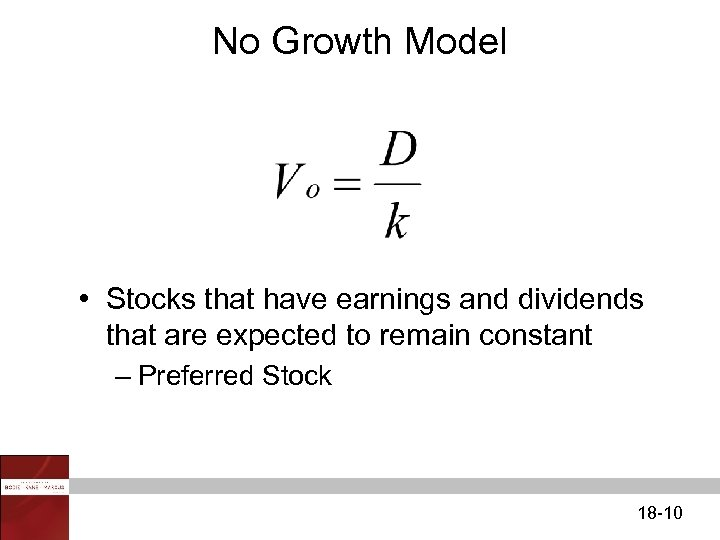No Growth Model • Stocks that have earnings and dividends that are expected to remain constant – Preferred Stock 18 -10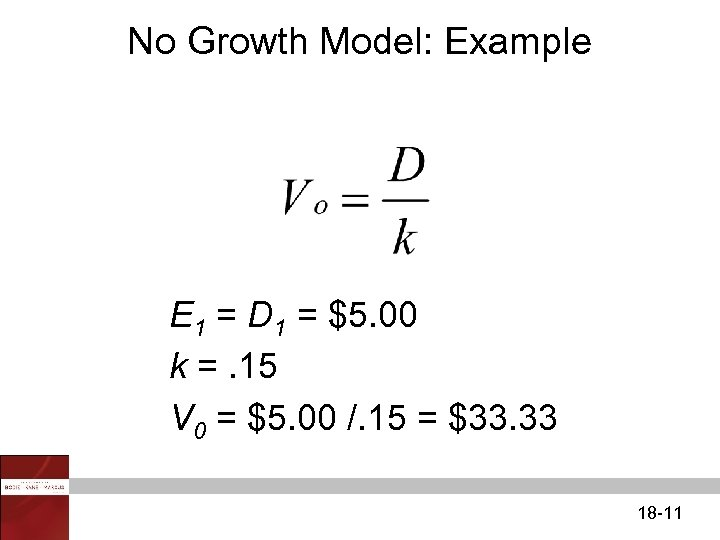No Growth Model: Example E 1 = D 1 = \$5. 00 k =. 15 V 0 = \$5. 00 /. 15 = \$33. 33 18 -11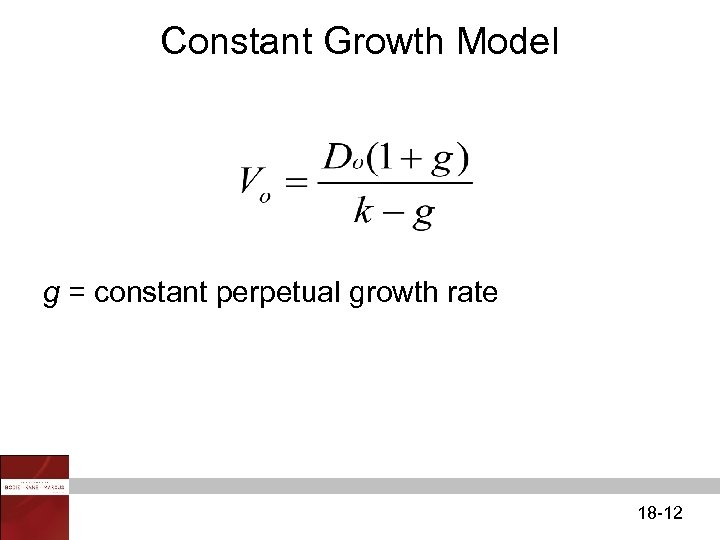Constant Growth Model g = constant perpetual growth rate 18 -12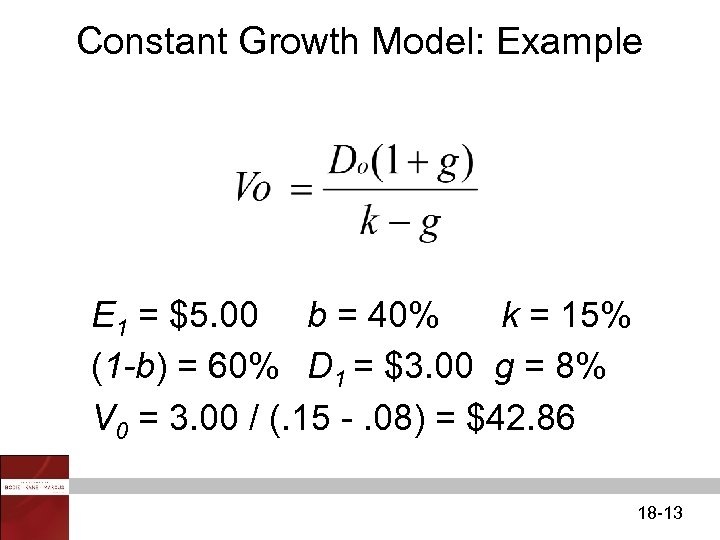Constant Growth Model: Example E 1 = \$5. 00 b = 40% k = 15% (1 -b) = 60% D 1 = \$3. 00 g = 8% V 0 = 3. 00 / (. 15 -. 08) = \$42. 86 18 -13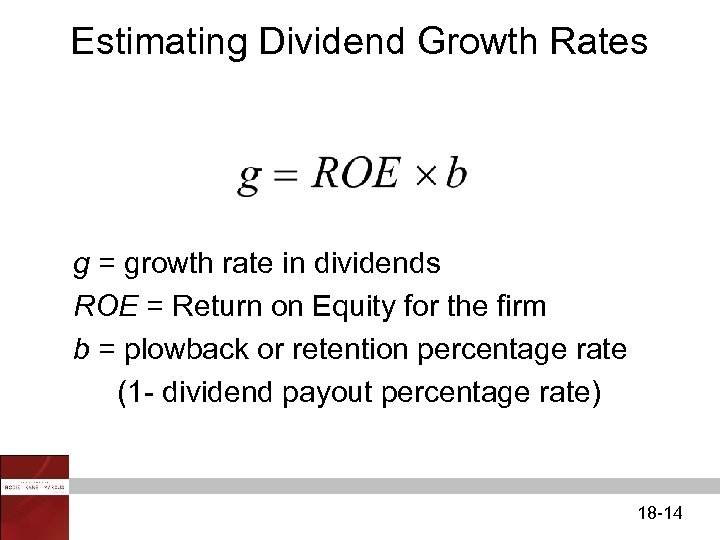Estimating Dividend Growth Rates g = growth rate in dividends ROE = Return on Equity for the firm b = plowback or retention percentage rate (1 - dividend payout percentage rate) 18 -14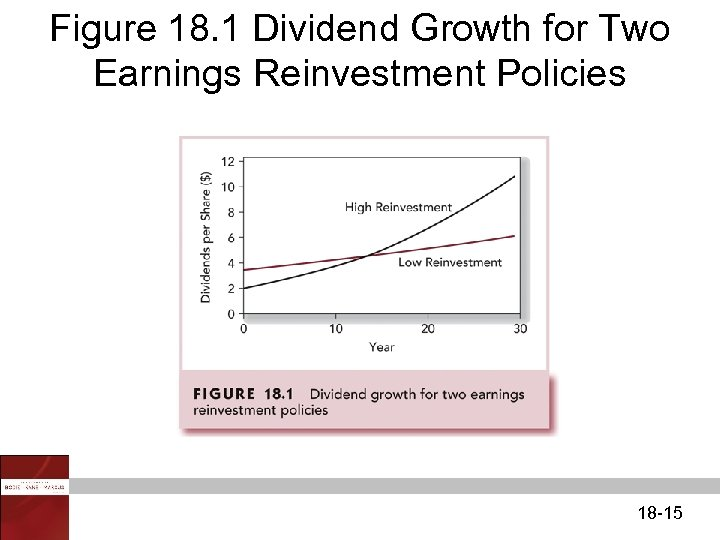Figure 18. 1 Dividend Growth for Two Earnings Reinvestment Policies 18 -15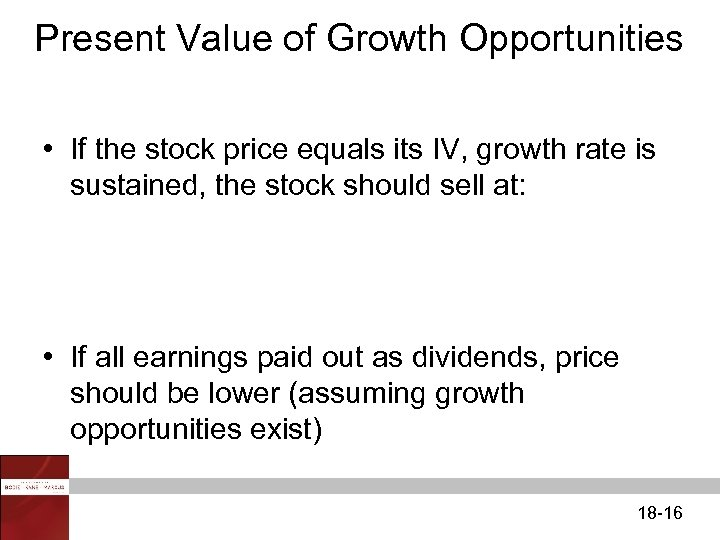Present Value of Growth Opportunities • If the stock price equals its IV, growth rate is sustained, the stock should sell at: • If all earnings paid out as dividends, price should be lower (assuming growth opportunities exist) 18 -16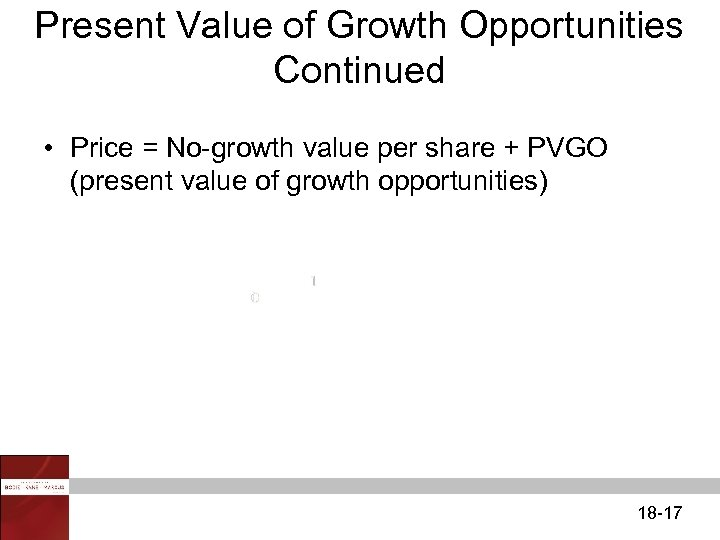Present Value of Growth Opportunities Continued • Price = No-growth value per share + PVGO (present value of growth opportunities) 18 -17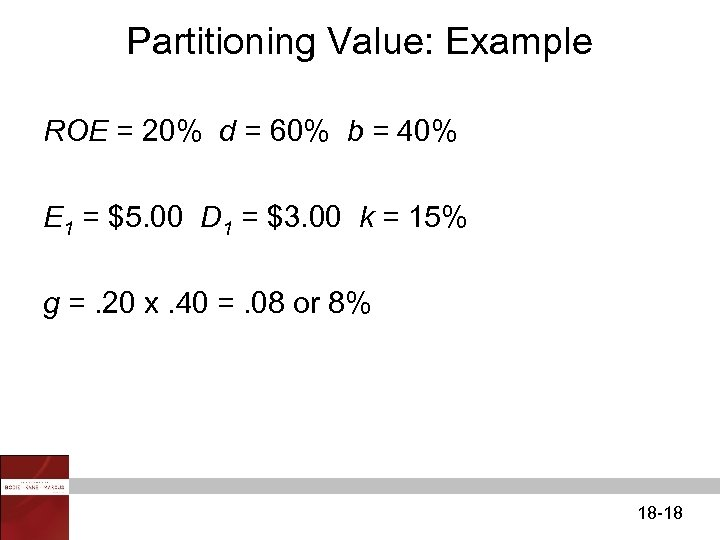Partitioning Value: Example ROE = 20% d = 60% b = 40% E 1 = \$5. 00 D 1 = \$3. 00 k = 15% g =. 20 x. 40 =. 08 or 8% 18 -18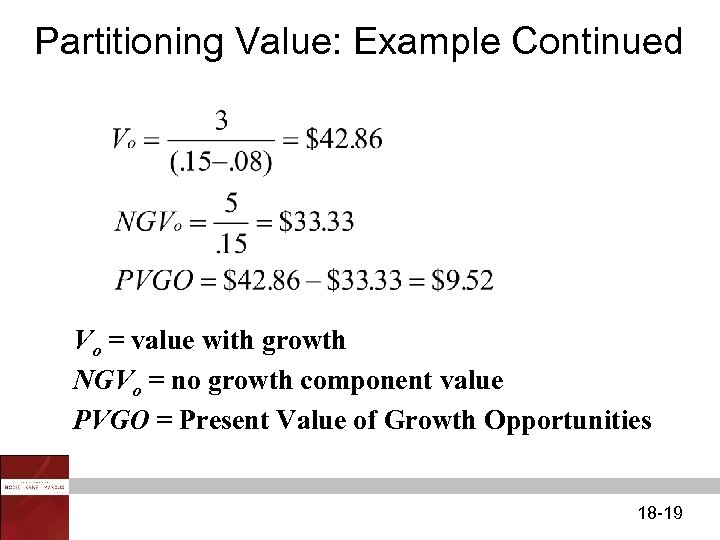Partitioning Value: Example Continued Vo = value with growth NGVo = no growth component value PVGO = Present Value of Growth Opportunities 18 -19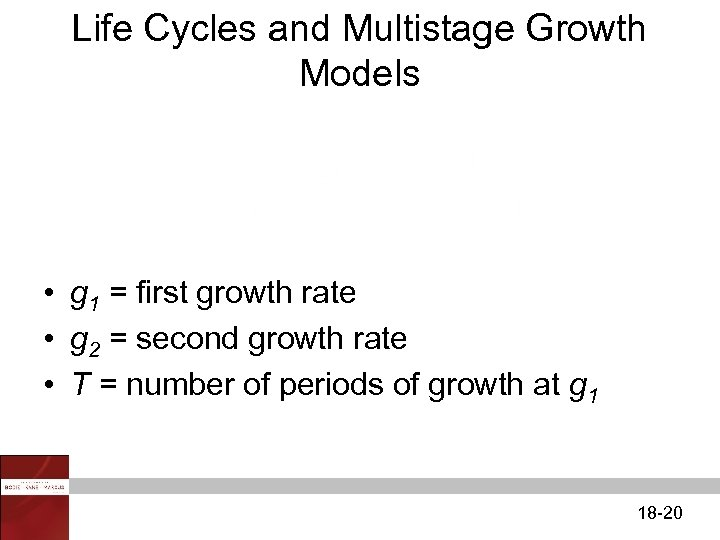Life Cycles and Multistage Growth Models • g 1 = first growth rate • g 2 = second growth rate • T = number of periods of growth at g 1 18 -20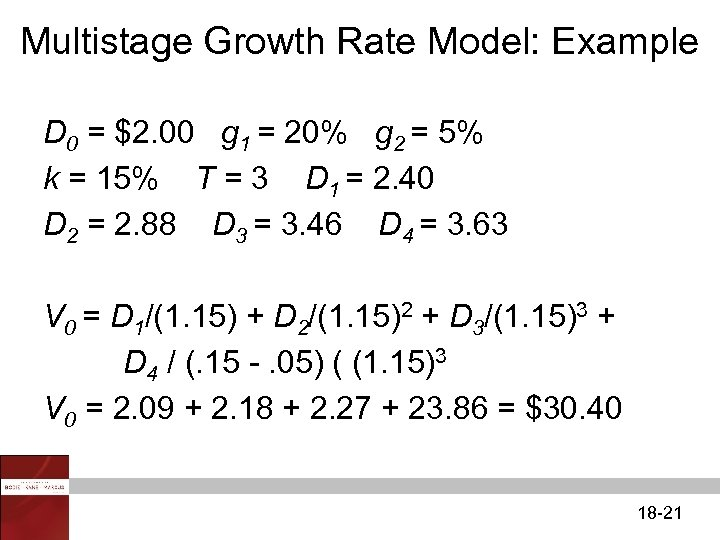Multistage Growth Rate Model: Example D 0 = \$2. 00 g 1 = 20% g 2 = 5% k = 15% T = 3 D 1 = 2. 40 D 2 = 2. 88 D 3 = 3. 46 D 4 = 3. 63 V 0 = D 1/(1. 15) + D 2/(1. 15)2 + D 3/(1. 15)3 + D 4 / (. 15 -. 05) ( (1. 15)3 V 0 = 2. 09 + 2. 18 + 2. 27 + 23. 86 = \$30. 40 18 -21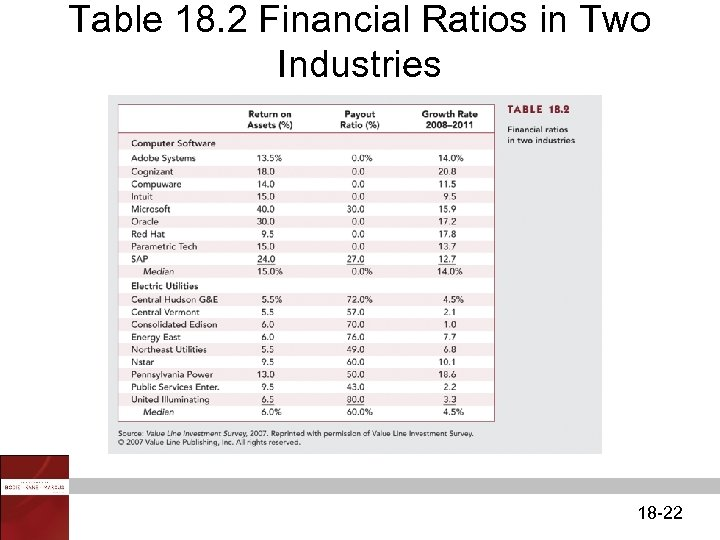Table 18. 2 Financial Ratios in Two Industries 18 -22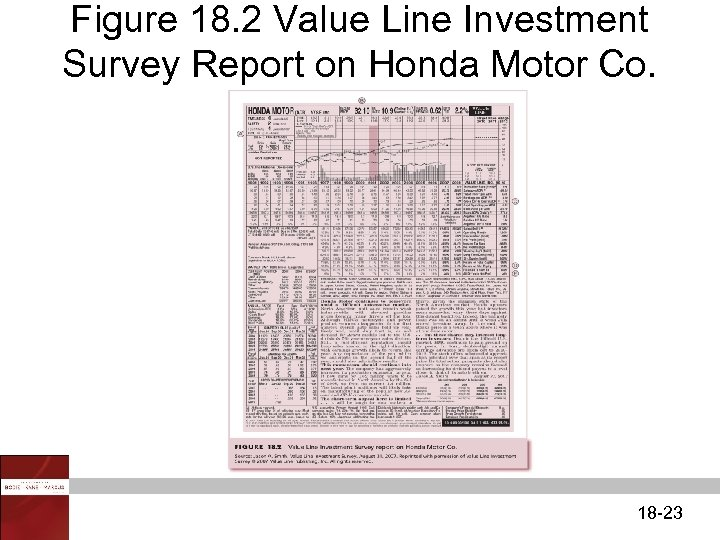Figure 18. 2 Value Line Investment Survey Report on Honda Motor Co. 18 -23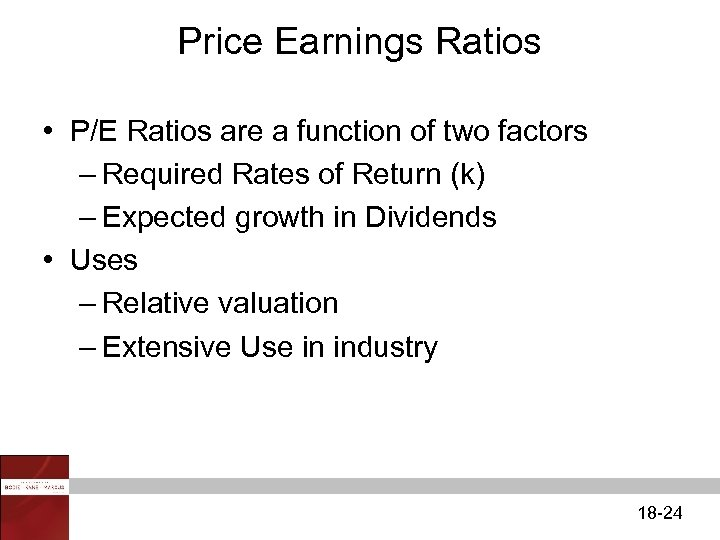Price Earnings Ratios • P/E Ratios are a function of two factors – Required Rates of Return (k) – Expected growth in Dividends • Uses – Relative valuation – Extensive Use in industry 18 -24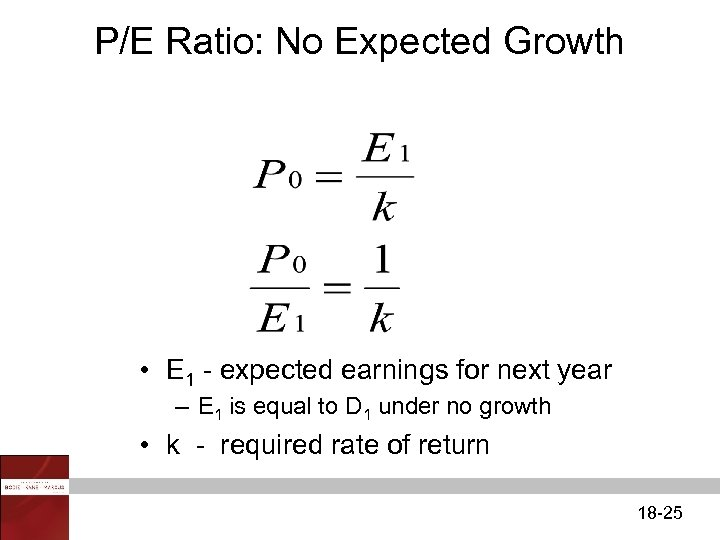P/E Ratio: No Expected Growth • E 1 - expected earnings for next year – E 1 is equal to D 1 under no growth • k - required rate of return 18 -25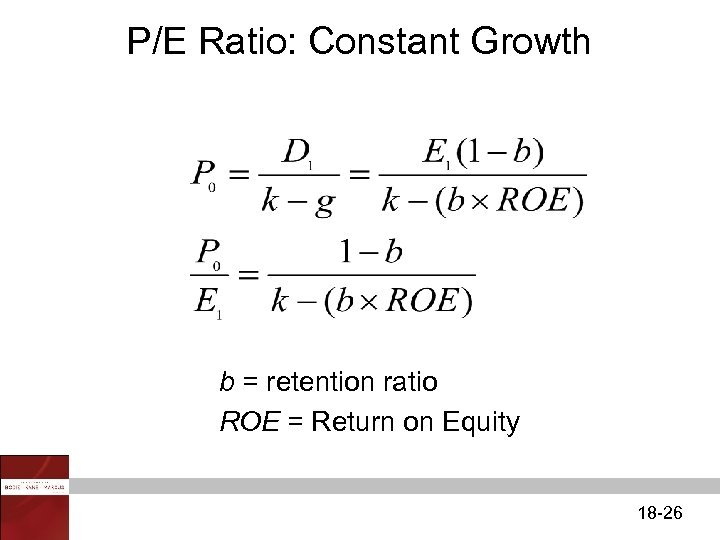P/E Ratio: Constant Growth b = retention ratio ROE = Return on Equity 18 -26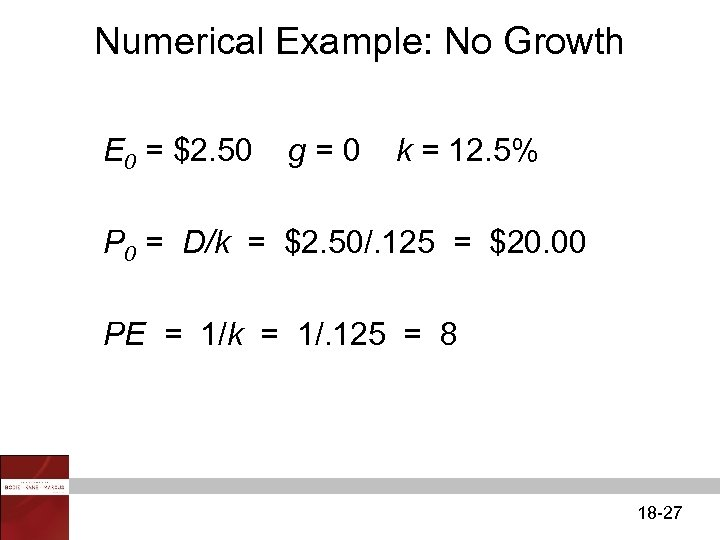Numerical Example: No Growth E 0 = \$2. 50 g=0 k = 12. 5% P 0 = D/k = \$2. 50/. 125 = \$20. 00 PE = 1/k = 1/. 125 = 8 18 -27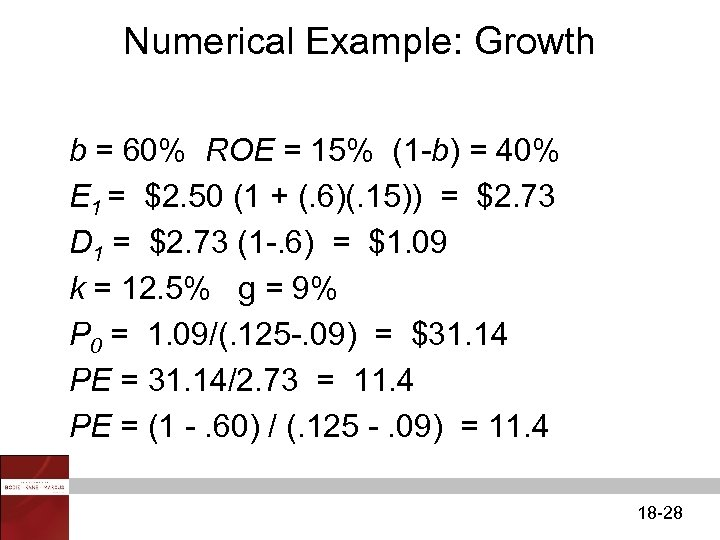Numerical Example: Growth b = 60% ROE = 15% (1 -b) = 40% E 1 = \$2. 50 (1 + (. 6)(. 15)) = \$2. 73 D 1 = \$2. 73 (1 -. 6) = \$1. 09 k = 12. 5% g = 9% P 0 = 1. 09/(. 125 -. 09) = \$31. 14 PE = 31. 14/2. 73 = 11. 4 PE = (1 -. 60) / (. 125 -. 09) = 11. 4 18 -28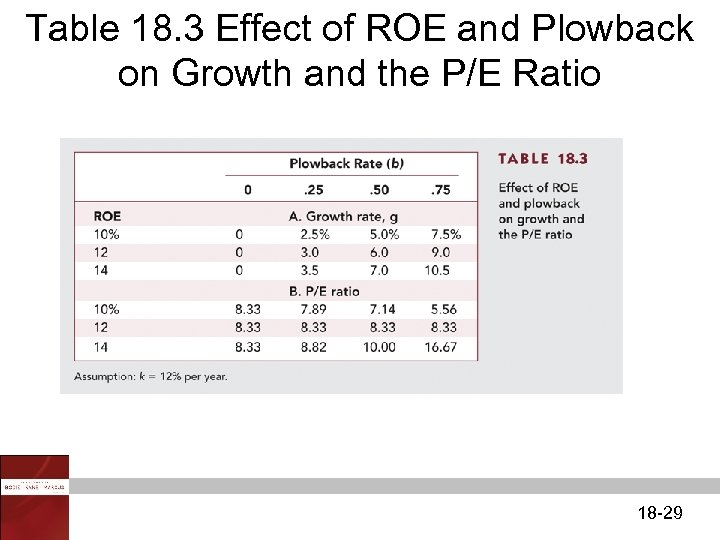Table 18. 3 Effect of ROE and Plowback on Growth and the P/E Ratio 18 -29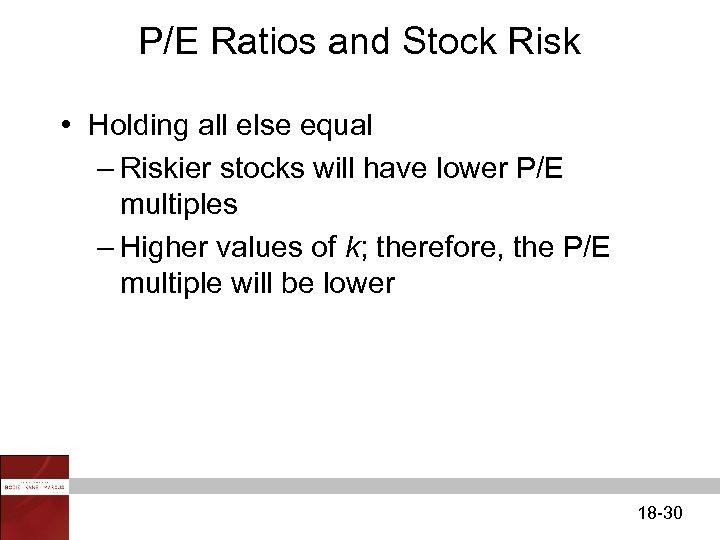P/E Ratios and Stock Risk • Holding all else equal – Riskier stocks will have lower P/E multiples – Higher values of k; therefore, the P/E multiple will be lower 18 -30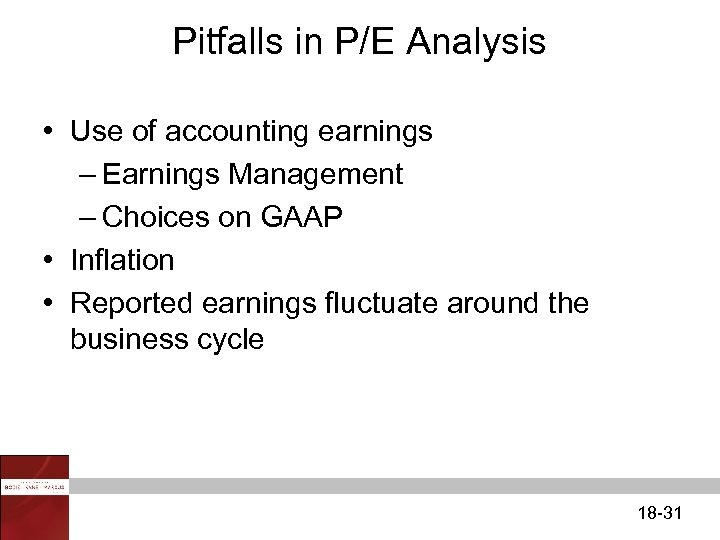Pitfalls in P/E Analysis • Use of accounting earnings – Earnings Management – Choices on GAAP • Inflation • Reported earnings fluctuate around the business cycle 18 -31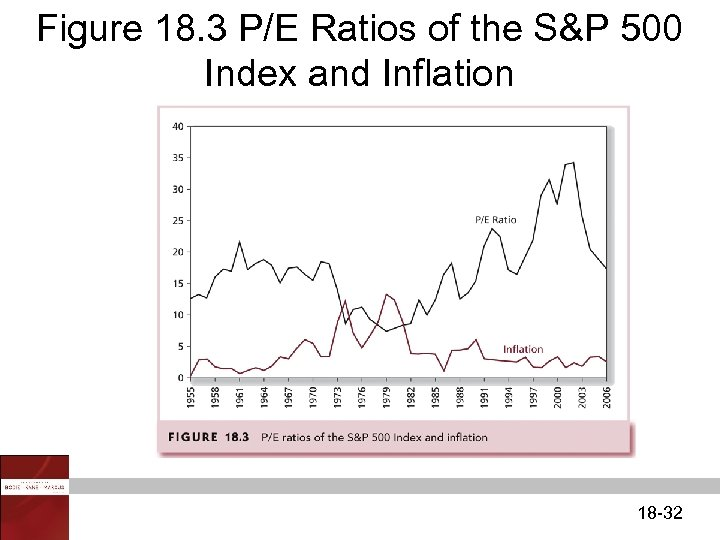Figure 18. 3 P/E Ratios of the S&P 500 Index and Inflation 18 -32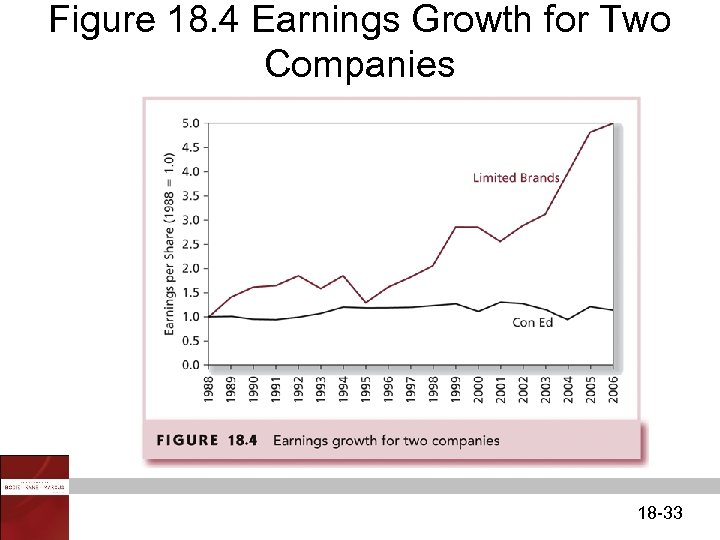Figure 18. 4 Earnings Growth for Two Companies 18 -33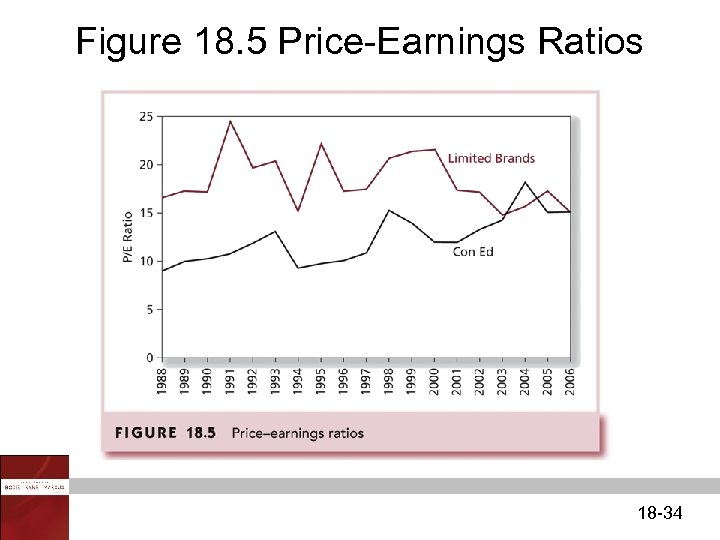Figure 18. 5 Price-Earnings Ratios 18 -34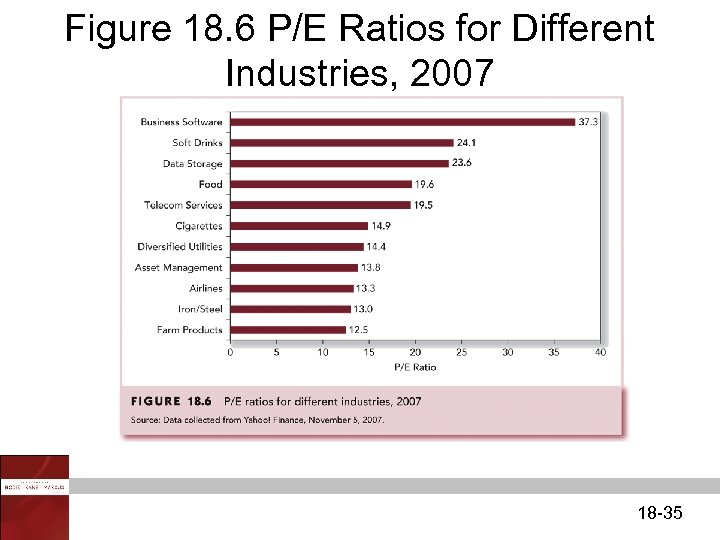Figure 18. 6 P/E Ratios for Different Industries, 2007 18 -35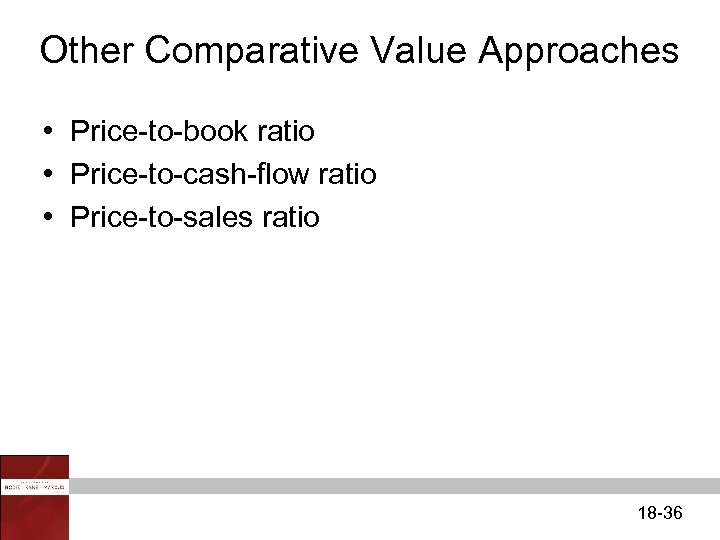Other Comparative Value Approaches • Price-to-book ratio • Price-to-cash-flow ratio • Price-to-sales ratio 18 -36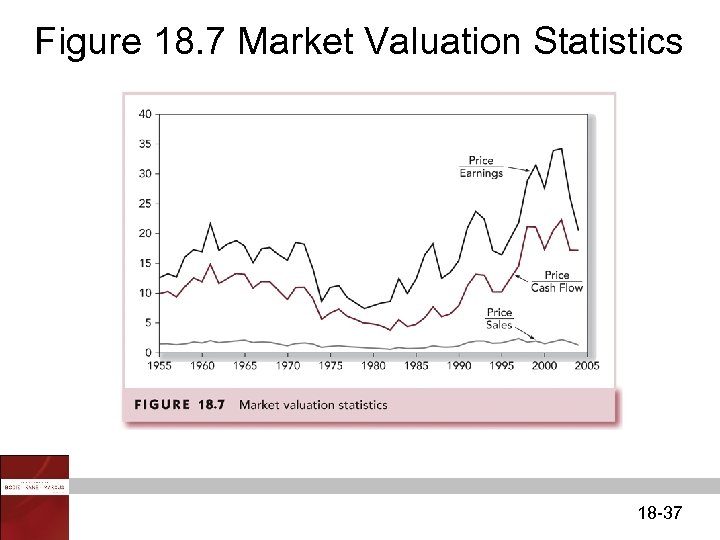Figure 18. 7 Market Valuation Statistics 18 -37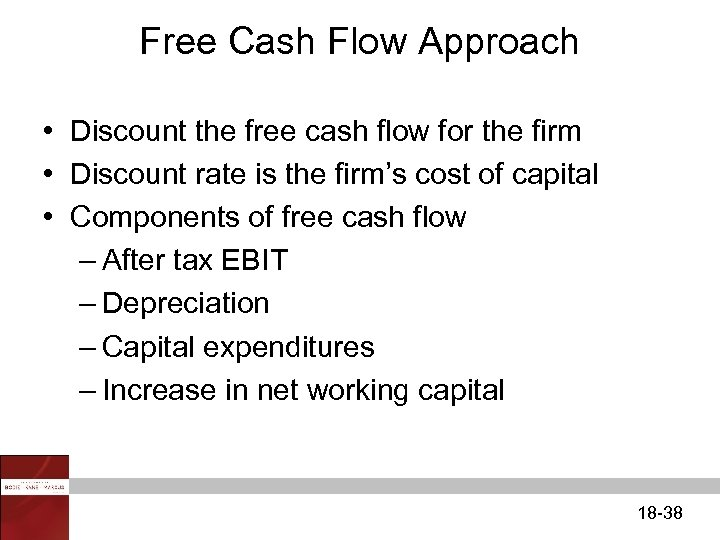Free Cash Flow Approach • Discount the free cash flow for the firm • Discount rate is the firm’s cost of capital • Components of free cash flow – After tax EBIT – Depreciation – Capital expenditures – Increase in net working capital 18 -38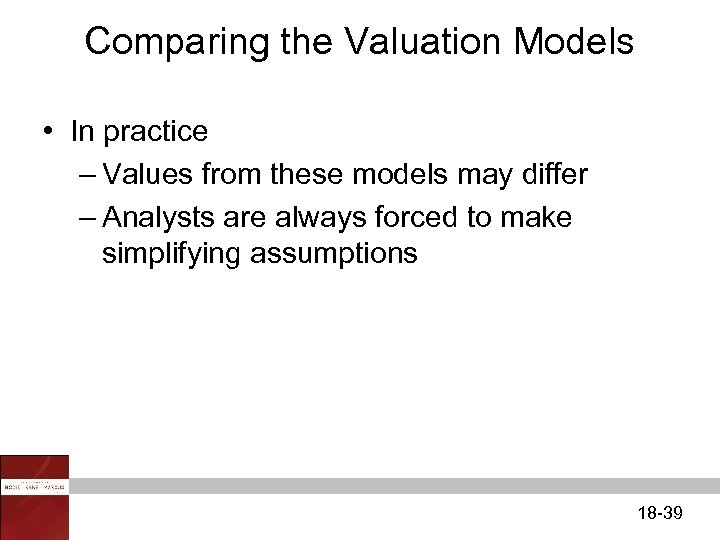Comparing the Valuation Models • In practice – Values from these models may differ – Analysts are always forced to make simplifying assumptions 18 -39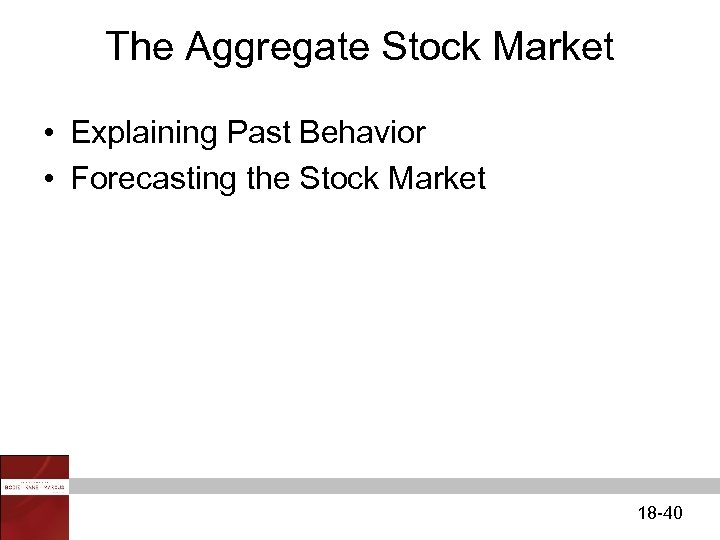The Aggregate Stock Market • Explaining Past Behavior • Forecasting the Stock Market 18 -40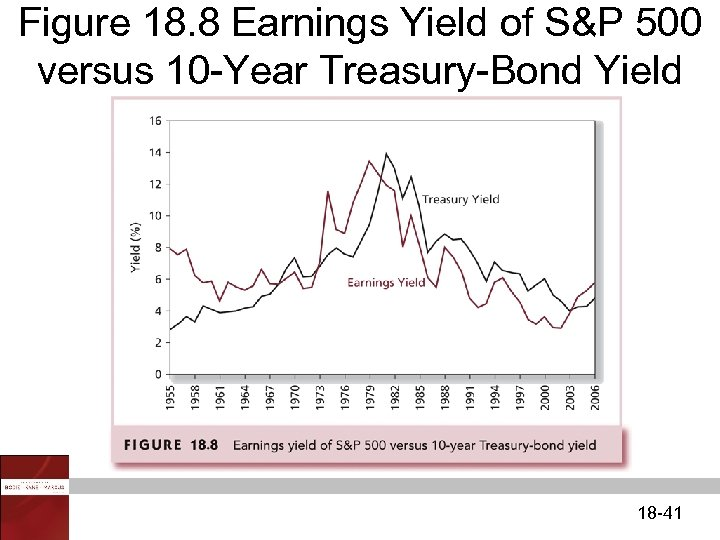Figure 18. 8 Earnings Yield of S&P 500 versus 10 -Year Treasury-Bond Yield 18 -41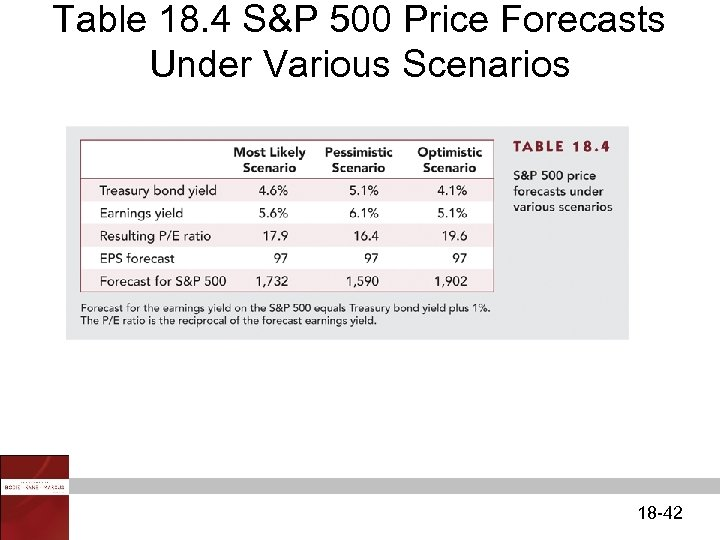Table 18. 4 S&P 500 Price Forecasts Under Various Scenarios 18 -42